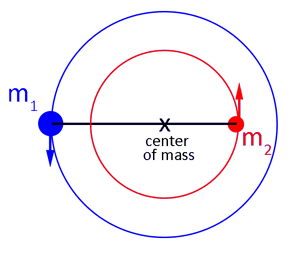# Kepler's 3rd law and a binary system

## Homework Statement

I am having a issue with the question, when I check the solution to the problem, I cant seem to make sense of how they have derived there equation.

Q: By analyzing the superposition of frequencies and amplitudes in the radial velocity data for a star 55 Cancri A, the first three exoplanets in the system were deduced. The star was found ti have the following observed parameters: 55 Cancri b corresponds to ##V_{obs}=71.8ms^m{-s}## and a ##P=14.6## days, 55 Cancri c corresponds to ##V_{obs}=10.0ms^{-s}##, ##P=43.9## days and 55 Cancri d corresponds to ##V_{obs}=47.2^m{-s}##, and ##P=5218## days. Assuming the orbits are coplanar and circular, determin, (i) which planet is furthest from the star, (ii) which planet has the lowest mass and (iii) which planet has the highest mass.

There equation for the solution is

$$M_p=\left(\frac{PM_s^2}{2\pi G}\right)\cdot \frac{V_{\left\{obs\right\}\:}}{sin\left(i\right)\:}$$

## Homework Equations

Mass function
##\frac{PV_{obs}^3}{2\pi G}=\frac{M_2sin^3\left(i\right)}{\left(M_1+M_2\right)}##

## The Attempt at a Solution[/B]
I am slightly confused by there solution because if I were to rearrange the equation mass function to make ##M_2## the subject I get the following

##M_2=\left(\frac{PM_1^2}{2\pi G}\right)^{\frac{1}{3}}\cdot \frac{V_{obs}}{sin\left(i\right)}##

which it the same as the equation given in the solution, but my assumption is that ##M_1>>M_2##

but this contradicts the diagram that I have shown because m1 is orbiting m2 which make m2 the larger of the two stars. This make me think maybe I am misunderstanding either the question, or the physics.